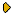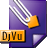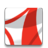Журнал Экспериментальной и Теоретической Физики
 НАЧАЛО | ПОИСК | ДЛЯ АВТОРОВ | ПОМОЩЬОбщая информация о журналеЗолотые страницыАдреса редакцииСодержание журналаСообщения редакцииПравила для авторовЗагрузить статьюПроверить статус статьиЖЭТФ, Том 146, Вып. 2, стр. 352 (Август 2014)
(Английский перевод - JETP, Vol. 119, No 2, p. 311, August 2014 доступен on-line на www.springer.com )

NONLINEAR DYNAMICS OF MAGNETOHYDRODYNAMIC FLOWS OF HEAVY FLUID ON SLOPE IN SHALLOW WATER APPROXIMATION

Поступила в редакцию: 8 Октября 2013

DOI: 10.7868/S0044451014080124DJVU (188.7K)PDF (313K)

Magnetohydrodynamic equations for a heavy fluid over an arbitrary surface are studied in the shallow water approximation. While solutions to the shallow water equations for a neutral fluid are well known, shallow water magnetohydrodynamic (SMHD) equations over a nonflat boundary have an additional dependence on the magnetic field, and the number of equations in the magnetic case exceeds that in the neutral case. As a consequence, the number of Riemann invariants defining SMHD equations is also greater. The classical simple wave solutions do not exist for hyperbolic SMHD equations over an arbitrary surface due to the appearance of a source term. In this paper, we suggest a more general definition of simple wave solutions that reduce to the classical ones in the case of zero source term. We show that simple wave solutions exist only for underlying surfaces that are slopes of constant inclination. All self-similar discontinuous and continuous solutions are found. Exact explicit solutions of the initial discontinuity decay problem over a slope are found. It is shown that the initial discontinuity decay solution is represented by one of four possible wave configurations. For each configuration, the necessary and sufficient conditions for its realization are found. The change of dependent and independent variables transforming the initial equations over a slope to those over a flat plane is found.

Сообщить о технических проблемах